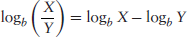CHAPTER 1

Some Basic Math

In this chapter we will review three math topics—logarithms, combinatorics, and geometric series—and one financial topic, discount factors. Emphasis will be given to the specific aspects of these topics that are most relevant to risk management.

LOGARITHMS

In mathematics, logarithms, or logs, are related to exponents, as follows:

(1.1)We say, “The log of a, base b, equals x, which implies that a equals b to the x and vice versa.” If we take the log of the right-hand side of Equation 1.1 and use the identity from the left-hand side of the equation, we can show that:

(1.2)Taking the log of bx effectively cancels out the exponentiation, leaving us with x.

An important property of logarithms is that the logarithm of the product of two variables is equal to the sum of the logarithms of those two variables. For two variables, X and Y:

(1.3)Similarly, the logarithm of the ratio of two variables is equal to the difference of their logarithms:

(1.4)If we replace Y with X in Equation 1.3, we get:

(1.5)

We can generalize this result to get the following power ...

Get Mathematics and Statistics for Financial Risk Management now with the O’Reilly learning platform.

O’Reilly members experience books, live events, courses curated by job role, and more from O’Reilly and nearly 200 top publishers.